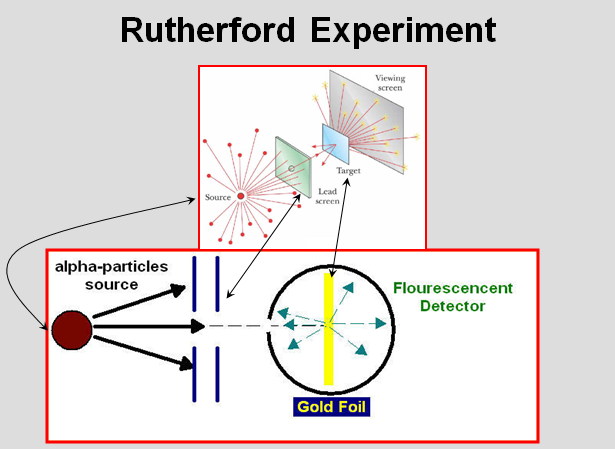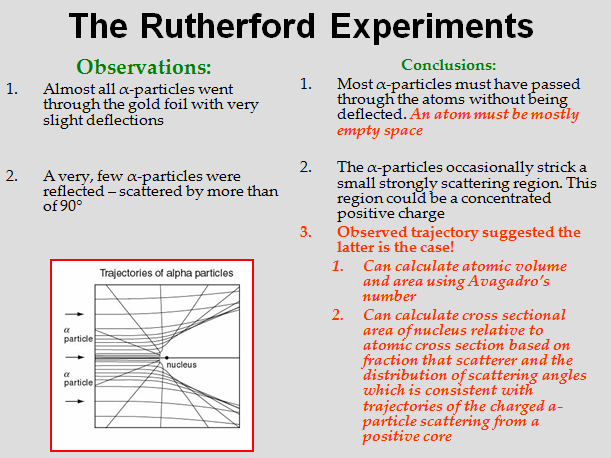# SCC Education

## Atomic structure

Atomic structure
Black body Radiation and Photoelectric Effect gave the impulse to understand the nature of light
However, there was no understanding on how electrons “exist” inside matter
It was known that atoms that form matter contain electrons (Thompson, 1897) and that atoms are neutral

Thomson Model
Electrons are embedded within spherically distributed, positive charge (so-called “plum pudding” model)
Both the positive charge and the mass of the atom would be more or less uniformly distributed over its size
Approximately 10-10 meters across = 1 Å = 0.1 nm
Problems with Thomson’s Model
How does the atom emit radiation?
This model soon came into conflict with experiments by Rutherford

The Rutherford Experiments
Rutherford discovered α (alpha)-particles
α-particles are the nuclei of helium atoms, which were
products of nuclear disintegration
He used α-particles in various studies
In 1909, he with co-workers (Hans Geiger and Ernest
Marsden) experimented with streams of alpha particles
passing through a thin gold foil
The foil thickness was ~ 8.6 x 10-6 cm
The foil was thin and so was supported on a glass plate
The plate itself was found to be transparent to the alpha particlesSince atoms have no overall electrical charge (atoms
are neutral), there must be just enough negatively
charged particles outside the nucleus to just balance
the positively-charged nucleus
Therefore,
The Rutherford's experiment suggested that the atom
consists of mostly empty space with a very small
positively-charged nucleus, outside which are just
enough negative charges to equal the positive charge in
the nucleus
Since the electrons do not crash into the nucleus but
exist in stable atoms, this suggests a planetary model
with negatively charged electrons circulating around a
positive core
Estimate of Nuclear Cross Section
Approximately one in 104 a particles with energies of
~ 107 eV are scattered backward from a one micron
thick sheet of metal foil
If atomic spacing is ~ 1 A, then there are 104 atomic
layers so the probability of scattering by a single layers
is 10-8
This implies the nuclear cross section is one part in
108 of the atomic cross section

Since the area goes as the square of the radius the
nuclear radius is smaller than atomic radius by a factor
of 10-4
Since the atomic diameter is ~ 1 A, the nuclear
diameter is ~ 10-4 A
The model of a small positively charge nuclear core
was confirmed by a comparison with the angular
distribution to scattered a-particles
The incident a-particles are assumed to have a
uniform distribution in space. Initial trajectories that are
headed towards the nuclei of atoms in the foil with
random separations from the nearest nuclear core
Full trajectory calculated for each distance of the
trajectory line from the center of the nearest nucleus to
give the scattering angle
From the distribution of distances of the a-particles
from the positive core calculate distribution of
scattering angle
Agreement found with measured scattering angle
distribution